# NF-ResNet：去掉BN歸一化，值得細讀的網路訊號分析 | ICLR 2021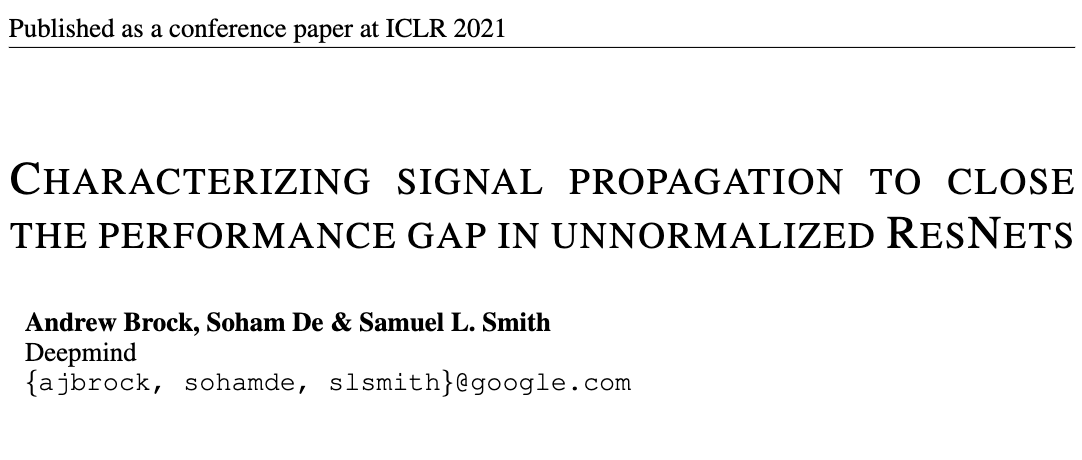# Introduction

BatchNorm是深度學習中核心計算元件，大部分的SOTA影象模型都使用它，主要有以下幾個優點： * 平滑損失曲線，可使用更大的學習率進行學習。 * 根據minibatch計算的統計資訊相當於為當前的batch引入噪聲，有正則化作用，防止過擬合。 * 在初始階段，約束殘差分支的權值，保證深度殘差網路有很好的資訊傳遞，可訓練超深的網路。

然而，儘管BatchNorm很好，但還是有以下缺點： * 效能受batch size影響大，batch size小時表現很差。 * 帶來訓練和推理時用法不一致的問題。 * 增加記憶體消耗。 * 實現模型時常見的錯誤來源，特別是分散式訓練。 * 由於精度問題，難以在不同的硬體上覆現訓練結果。

目前，很多研究開始尋找替代BatchNorm的歸一化層，但這些替代層要麼表現不行，要麼會帶來新的問題，比如增加推理的計算消耗。而另外一些研究則嘗試去掉歸一化層，比如初始化殘差分支的權值，使其輸出為零，保證訓練初期大部分的資訊通過skip path進行傳遞。雖然能夠訓練很深的網路，但使用簡單的初始化方法的網路的準確率較差，而且這樣的初始化很難用於更復雜的網路中。
因此，論文希望找出一種有效地訓練不含BatchNorm的深度殘差網路的方法，而且測試集效能能夠媲美當前的SOTA，論文主要貢獻如下： * 提出訊號傳播圖(Signal Propagation Plots, SPPs)，可輔助觀察初始階段的推理訊號傳播情況，確定如何設計無BatchNorm的ResNet來達到類似的訊號傳播效果。 * 驗證發現無BatchNorm的ResNet效果不好的關鍵在於非線性啟用(ReLU)的使用，經過非線性啟用的輸出的均值總是正數，導致權值的均值隨著網路深度的增加而急劇增加。於是提出Scaled Weight Standardization，能夠阻止訊號均值的增長，大幅提升效能。 * 對ResNet進行normalization-free改造以及新增Scaled Weight Standardization訓練，在ImageNet上與原版的ResNet有相當的效能，層數達到288層。 * 對RegNet進行normalization-free改造，結合EfficientNet的混合縮放，構造了NF-RegNet系列，在不同的計算量上都達到與EfficientNet相當的效能。

# Signal Propagation Plots

許多研究從理論上分析ResNet的訊號傳播，卻很少會在設計或魔改網路的時候實地驗證不同層數的特徵縮放情況。實際上，用任意輸入進行前向推理，然後記錄網路不同位置特徵的統計資訊，可以很直觀地瞭解資訊傳播狀況並儘快發現隱藏的問題，不用經歷漫長的失敗訓練。於是，論文提出了訊號傳播圖(Signal Propagation Plots,SPPs)，輸入隨機高斯輸入或真實訓練樣本，然後分別統計每個殘差block輸出的以下資訊：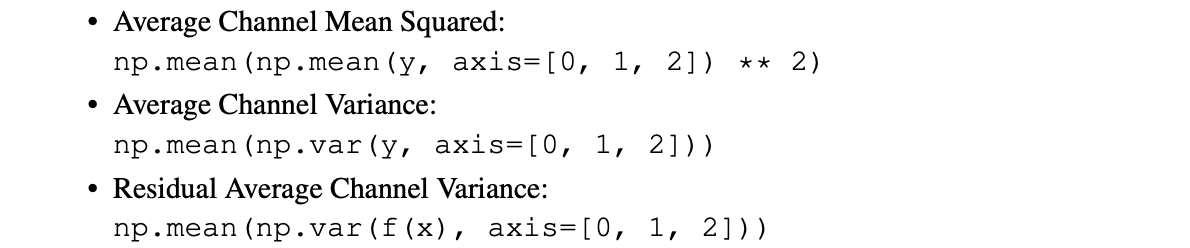• Average Channel Squared Mean，在NHW維計算均值的平方(平衡正負均值)，然後在C維計算平均值，越接近零是越好的。
• Average Channel Variance，在NHW維計算方差，然後在C維計算平均值，用於衡量訊號的幅度，可以看到訊號是爆炸抑或是衰減。
• Residual Average Channel Variance，僅計算殘差分支輸出，用於評估分支是否被正確初始化。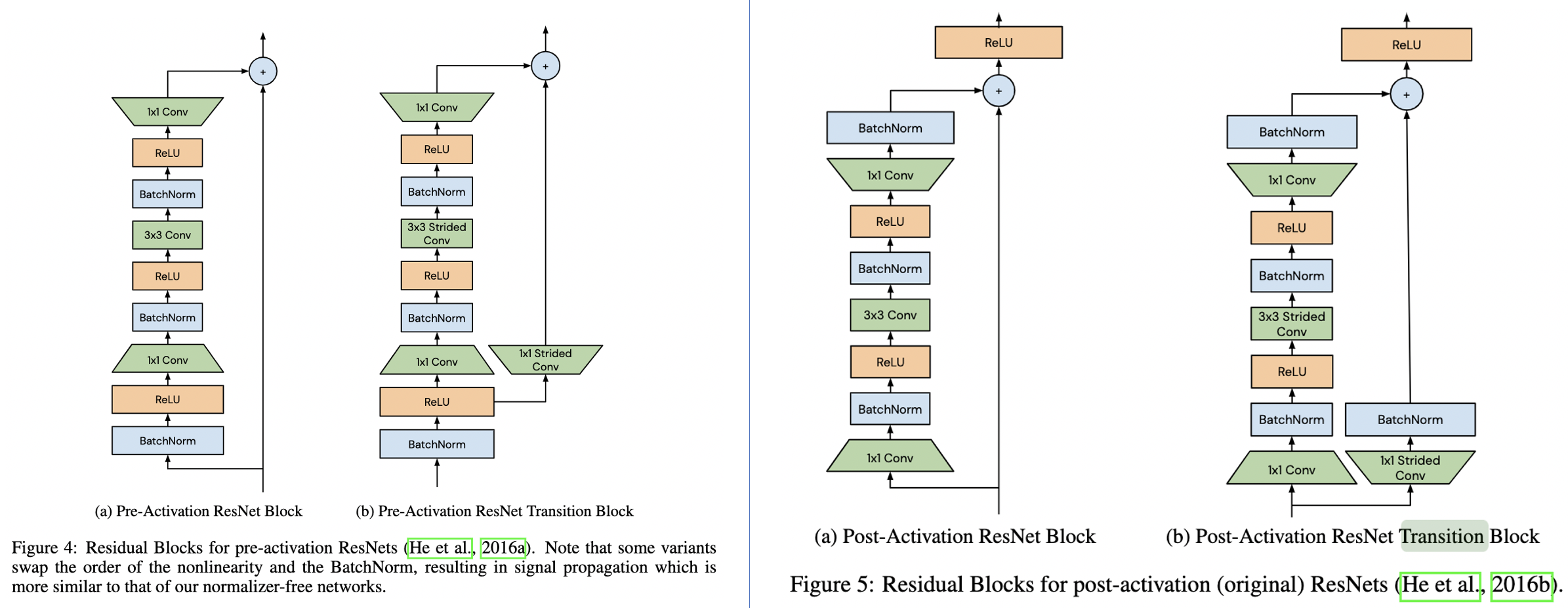論文對常見的BN-ReLU-Conv結構和不常見的ReLU-BN-Conv結構進行了實驗統計，實驗的網路為600層ResNet，採用He初始化，定義residual block為$x_{l+1}=f_{l}(x_{l}) + x_{l}$，從SPPs可以發現了以下現象：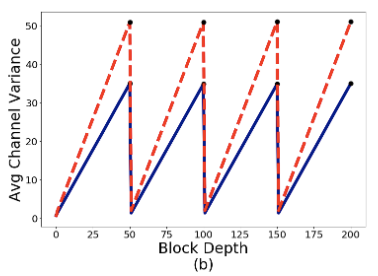• Average Channel Variance隨著網路深度線性增長，然後在transition block處重置為較低值。這是由於在訓練初始階段，residual block的輸出的方差為$Var(x_{l+1})=Var(f_{l}(x_{l})) + Var(x_{l})$，不斷累積residual branch和skip path的方差。而在transition block處，skip path的輸入被BatchNorm處理過，所以block的輸出的方差直接被重置了。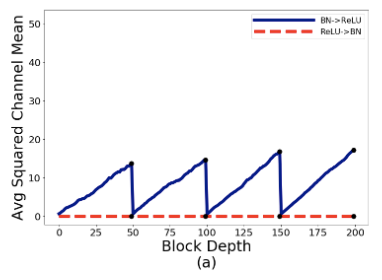• BN-ReLU-Conv的Average Squared Channel Means也是隨著網路深度不斷增加，雖然BatchNorm的輸出是零均值的，但經過ReLU之後就變成了正均值，再與skip path相加就不斷地增加直到transition block的出現，這種現象可稱為mean-shift。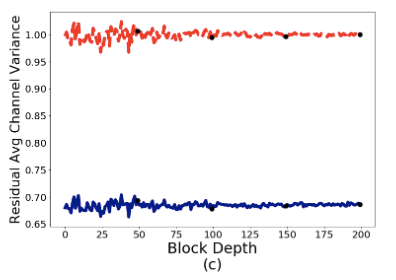• BN-ReLU的Residual Average Channel Variance大約為0.68，ReLU-BN的則大約為1。BN-ReLU的方差變小主要由於ReLU，後面會分析到，但理論應該是0.34左右，而且這裡每個transition block的殘差分支輸出卻為1，有點奇怪，如果知道的讀者麻煩評論或私信一下。

假如直接去掉BatchNorm，Average Squared Channel Means和Average Channel Variance將會不斷地增加，這也是深層網路難以訓練的原因。所以要去掉BatchNorm，必須設法模擬BatchNorm的訊號傳遞效果。

# Normalizer-Free ResNets(NF-ResNets)

根據前面的SPPs，論文設計了新的redsidual block$x_{l+1}=x_l+\alpha f_l(x_l/\beta_l)$，主要模擬BatchNorm在均值和方差上的表現，具體如下： * $f(\cdot)$為residual branch的計算函式，該函式需要特殊初始化，保證初期具有保持方差的功能，即$Var(f_l(z))=Var(z)$，這樣的約束能夠幫助更好地解釋和分析網路的訊號增長。 * $\beta_l=\sqrt{Var(x_l)}$為固定標量，值為輸入特徵的標準差，保證$f_l(\cdot)$為單位方差。 * $\alpha$為超引數，用於控制block間的方差增長速度。

根據上面的設計，給定$Var(x_0)=1$和$\beta_l=\sqrt{Var(x_l)}$，可根據$Var(x_l)=Var(x_{l-1})+\alpha^2$直接計算第$l$個residual block的輸出的方差。為了模擬ResNet中的累積方差在transition block處被重置，需要將transition block的skip path的輸入縮小為$x_l/\beta_l$，保證每個stage開頭的transition block輸出方差滿足$Var(x_{l+1})=1+\alpha^2$。將上述簡單縮放策略應用到殘差網路並去掉BatchNorm層，就得到了Normalizer-Free ResNets(NF-ResNets)。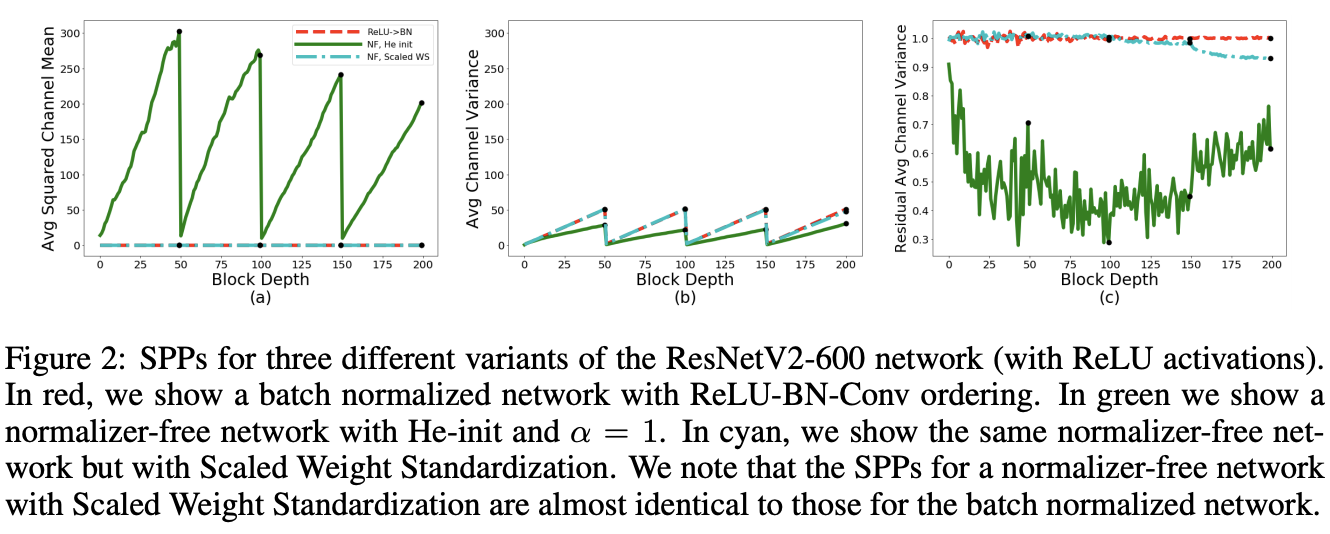### ReLU Activations Induce Mean Shifts

論文對使用He初始化的NF-ResNet進行SPPs分析，結果如圖2，發現了兩個比較意外的現象： * Average Channel Squared Mean隨著網路變深不斷增加，值大到超過了方差，有mean-shift現象。 * 跟BN-ReLU-Conv類似，殘差分支輸出的方差始終小於1。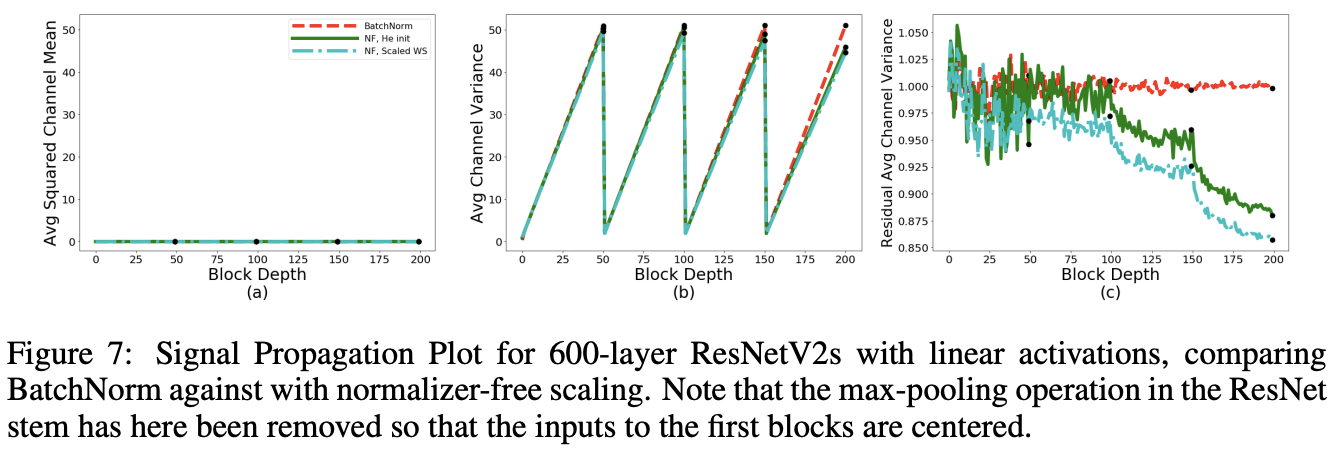為了驗證上述現象，論文將網路的ReLU去掉再進行SPPs分析。如圖7所示，當去掉ReLU後，Average Channel Squared Mean接近於0，而且殘差分支輸出的接近1，這表明是ReLU導致了mean-shift現象。
論文也從理論的角度分析了這一現象，首先定義轉化$z=Wg(x)$，$W$為任意且固定的矩陣，$g(\cdot)$為作用於獨立同分布輸入$x$上的elememt-wise啟用函式，所以$g(x)$也是獨立同分布的。假設每個維度$i$都有$\mathbb{E}(g(x_i))=\mu_g$以及$Var(g(x_i))=\sigma^2_g$，則輸出$z_i=\sum^N_jW_{i,j}g(x_j)$的均值和方差為：其中，$\mu w_{i,.}$和$\sigma w_{i,.}$為$W$的$i$行(fan-in)的均值和方差：當$g(\cdot)$為ReLU啟用函式時，則$g(x)\ge 0$，意味著後續的線性層的輸入都為正均值。如果$x_i\sim\mathcal{N}(0,1)$，則$\mu_g=1/\sqrt{2\pi}$。由於$\mu_g>0$，如果$\mu w_i$也是非零，則$z_i$同樣有非零均值。需要注意的是，即使$W$從均值為零的分佈中取樣而來，其實際的矩陣均值肯定不會為零，所以殘差分支的任意維度的輸出也不會為零，隨著網路深度的增加，越來越難訓練。

### Scaled Weight Standardization

為了消除mean-shift現象以及保證殘差分支$f_l(\cdot)$具有方差不變的特性，論文借鑑了Weight Standardization和Centered Weight Standardization，提出Scaled Weight Standardization(Scaled WS)方法，該方法對卷積層的權值重新進行如下的初始化：$\mu$和$\sigma$為卷積核的fan-in的均值和方差，權值$W$初始為高斯權值，$\gamma$為固定常量。代入公式1可以得出，對於$z=\hat{W}g(x)$，有$\mathbb{E}(z_i)=0$，去除了mean-shift現象。另外，方差變為$Var(z_i)=\gamma^2\sigma^2_g$，$\gamma$值由使用的啟用函式決定，可保持方差不變。
Scaled WS訓練時增加的開銷很少，而且與batch資料無關，在推理的時候更是無額外開銷的。另外，訓練和測試時的計算邏輯保持一致，對分散式訓練也很友好。從圖2的SPPs曲線可以看出，加入Scaled WS的NF-ResNet-600的表現跟ReLU-BN-Conv十分相似。

### Determining Nonlinerity-Specific Constants

最後的因素是$\gamma$值的確定，保證殘差分支輸出的方差在初始階段接近1。$\gamma$值由網路使用的非線性啟用型別決定，假設非線性的輸入$x\sim\mathcal{N}(0,1)$，則ReLU輸出$g(x)=max(x,0)$相當於從方差為$\sigma^2_g=(1/2)(1-(1/\pi))$的高斯分佈取樣而來。由於$Var(\hat{W}g(x))=\gamma^2\sigma^2_g$，可設定$\gamma=1/\sigma_g=\frac{\sqrt{2}}{\sqrt{1-\frac{1}{\pi}}}$來保證$Var(\hat{W}g(x))=1$。雖然真實的輸入不是完全符合$x\sim \mathcal{N}(0,1)$，在實踐中上述的$\gamma$設定依然有不錯的表現。
對於其他複雜的非線性啟用，如SiLU和Swish，公式推導會涉及複雜的積分，甚至推出不出來。在這種情況下，可使用數值近似的方法。先從高斯分佈中取樣多個$N$維向量$x$，計算每個向量的啟用輸出的實際方差$Var(g(x))$，再取實際方差均值的平方根即可。

### Other Building Block and Relaxed Constraints

本文的核心在於保持正確的資訊傳遞，所以許多常見的網路結構都要進行修改。如同選擇$\gamma$值一樣，可通過分析或實踐判斷必要的修改。比如SE模組$y=sigmoid(MLP(pool(h)))*h$，輸出需要與$[0,1]$的權值進行相乘，導致資訊傳遞減弱，網路變得不穩定。使用上面提到的數值近似進行單獨分析，發現期望方差為0.5，這意味著輸出需要乘以2來恢復正確的資訊傳遞。
實際上，有時相對簡單的網路結構修改就可以保持很好的資訊傳遞，而有時候即便網路結構不修改，網路本身也能夠對網路結構導致的資訊衰減有很好的魯棒性。因此，論文也嘗試在維持穩定訓練的前提下，測試Scaled WS層的約束的最大放鬆程度。比如，為Scaled WS層恢復一些卷積的表達能力，加入可學習的縮放因子和偏置，分別用於權值相乘和非線性輸出相加。當這些可學習引數沒有任何約束時，訓練的穩定性沒有受到很大的影響，反而對大於150層的網路訓練有一定的幫助。所以，NF-ResNet直接放鬆了約束，加入兩個可學習引數。
論文的附錄有詳細的網路實現細節，有興趣的可以去看看。

### Summary

總結一下，Normalizer-Free ResNet的核心有以下幾點： * 計算前向傳播的期望方差$\beta^2_l$，每經過一個殘差block穩定增加$\alpha^2$，殘差分支的輸入需要縮小$\beta_l$倍。 * 將transition block中skip path的卷積輸入縮小$\beta_l$倍，並在transition block後將方差重置為$\beta_{l+1}=1+\alpha^2$。 * 對所有的卷積層使用Scaled Weight Standardization初始化，基於$x\sim\mathcal{N}(0,1)$計算啟用函式$g(x)$對應的$\gamma$值，為啟用函式輸出的期望標準差的倒數$\frac{1}{\sqrt{Var(g(x))}}$。

# Experiments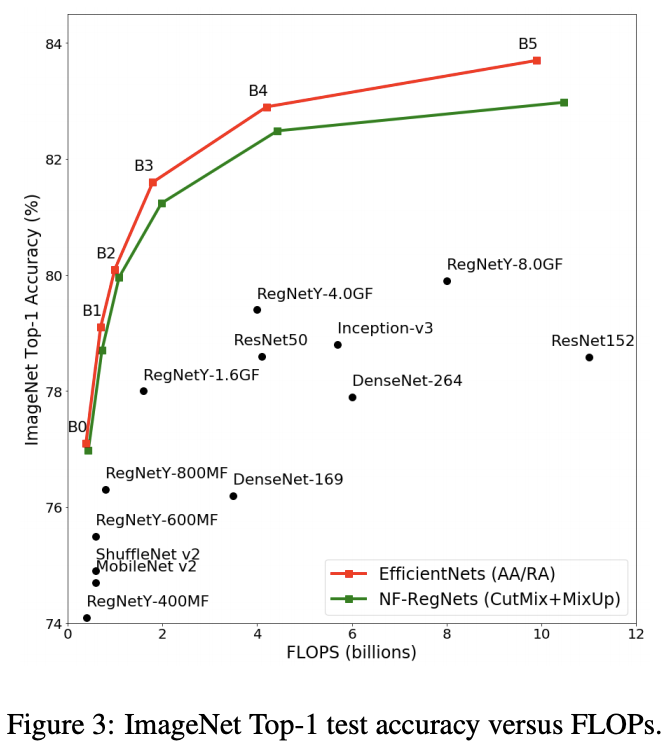對比RegNet的Normalizer-Free變種與其他方法的對比，相對於EfficientNet還是差點，但已經十分接近了。

# Conclusion

論文提出NF-ResNet，根據網路的實際訊號傳遞進行分析，模擬BatchNorm在均值和方差傳遞上的表現，進而代替BatchNorm。論文實驗和分析十分足，出來的效果也很不錯。一些初始化方法的理論效果是對的，但實際使用會有偏差，論文通過實踐分析發現了這一點進行補充，貫徹了實踐出真知的道理。